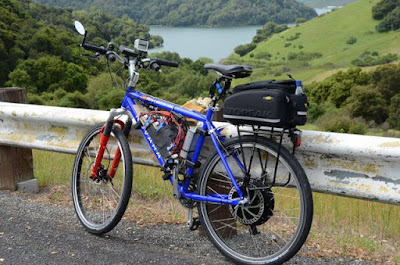### 800 mpg E-BikeSo what is the efficiency of a modern electric bike? How should we compare efficiency?The best way is to use cost. It is easy to do and easy to understand. What are the costs of electricity and gasoline?First of all, how much energy does an electric bike require? This depends on a number of factors like how much pedaling is done, how fast you are going and how hilly the terrain is. Let's assume little pedaling, since if you pedal a lot there might be no electricity use at all (in which case you are burning food which is MUCH more expensive than gasoline). For the situation of going at bicycle speeds of 15-20 miles per hour and no pedaling in flat to moderate hills the energy usage is generally around 20 watt hours per mile. For more hilly terrain it might go to 30 watt hours per mile. But how does this translate into miles per gallon equivalent?So what are the costs of gasoline and electricity? I'll pick generic figures for my area. Gasoline is close to \$4 per gallon and electricity is about \$0.20 per kilowatt hour. So if we spend \$4 we can buy one gallon of gas, or 20 kilowatt hours of electricity (that's 20,000 watt-hours). So how far can our 20 watt-hour per mile ebike go on four dollars, or the cost of one gallon of gas? If we ignore the losses of charging the battery, we could go 1,000 miles on a gallon's worth of dollars. Charging is pretty efficient, so deducting 20% will more than cover that, so the effective mileage of an ebike is about 800 miles per gallon. This is about 16 times better than the best car!So use an ebike for transportation when you can, and reduce the vehicle usage at least some. Every bit helps!Ride Safe!-- Alan, W6AKB